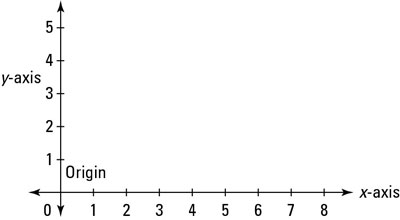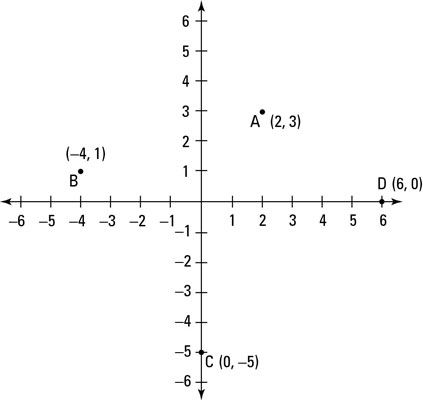##### Trigonometry Workbook For DummiesWhen math folks talk about using a graph, they’re usually referring to a Cartesian graph (also called the Cartesian coordinate system). The below figure shows an example of a Cartesian graph.A Cartesian graph includes horizontal and vertical axes, which cross at the origin (0,0).
A Cartesian graph is really just two number lines that cross at 0. These number lines are called the horizontal axis (also called the x-axis) and the vertical axis (also called the y-axis). The place where these two axes (plural of axis) cross is called the origin.

Plotting a point (finding and marking its location) on a graph isn’t much harder than finding a point on a number line, because a graph is just two number lines put together.

Every point on a Cartesian graph is represented by two numbers in parentheses, separated by a comma, called a set of coordinates. To plot any point, start at the origin, where the two axes cross. The first number tells you how far to go to the right (if positive) or left (if negative) along the horizontal axis. The second number tells you how far to go up (if positive) or down (if negative) along the vertical axis.

For example, here are the coordinates of four points called A, B, C, and D:

A = (2, 3) B = (–4, 1) C = (0, –5) D = (6, 0)The above figure depicts a graph with these four points plotted. Start at the origin, (0, 0). To plot point A, count 2 spaces to the right and 3 spaces up. To plot point B, count 4 spaces to the left (the negative direction) and then 1 space up. To plot point C, count 0 spaces left or right and then count 5 spaces down (the negative direction). And to plot point D, count 6 spaces to the right and then 0 spaces up or down.